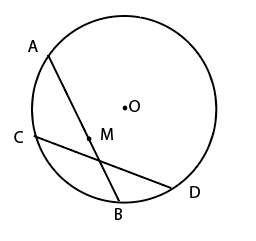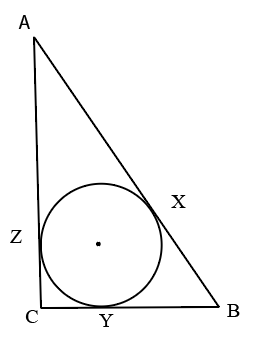# IIFT 2020

Instructions

For the following questions answer them individually

Question 11

# ABCD is a parallelogram whose diagonals are parallel to the lines $$2y—x—5=0$$ and $$y+2x—7=0$$ respectively.Then ABCDis -Question 12

# Suhani, an enterprising lady took the loan from M/s Koramattam Finance against her gold ornamentsat a simple interest of 12% per annum for 2 years. She, then, loaned 50% of the amount received fromM/s Koramattam Finance, to Vishamber at the rate of 16% per annum compounded half yearly for 2years and the remaining amount to Kalawati at the rate of 12% per annum compoundedannually for 2 years. What was the approximate percentage earning of Suhani at the end of 2 years ?Question 13

# A Financial Analyst estimates that the probability that the economy will experience recession in nextone year is 30%. He also believes that in case of recession, the probability that his mutual funds willincrease in value is 20%. He also believes that if there is no recession the probability that the value ofmutual funds will increase in value is 75%. Find the probability that mutualfunds value will increase.Question 14

# The probabilities of three mutually exclusive outcomes of anexperiment $$0.25(1 — x), 0.5(1 — 2x)$$ and $$0.25(1 + 4x)$$ respectively.Which of the following holds for the value of x ?Question 15

# Two cities Mathura and Agra, 48 kms apart, are located on the bank of River Yamuna. A motor boatgoes from Mathura to Agra and returns back as soon as possible. Yamuna flows at a speed of 6 km/hr.The motorboat completes the trip from Mathura to Agra and back in not more than 6 hours. Assumingthe motorboat does nothalt at Agra, what should be the minimum speed of motorboatin still water?Question 16

# A man, from the foot of the building P, walks towards the building Q. After walking for 2 mts, hefinds that buildings P and Q makean angle of elevation of $$60^{0}$$ and $$30^{0}$$ respectively. If building Q is1.5 mts high, find the distance between the tops of both buildings P and Q.Question 17

# At what time between 10.00 AM and 11.00 AM,the minute hand and the hour hand of a watch wouldmake an angle of $$180^{0}$$ ?Question 18

# An owner of a grocery shop purchases two varieties of grain. The price of first variety is twice the price of the second one. He mixes both the varieties and sells the mixture at the price of Rs. 28 per kg, making a profit of 25%. If the ratio of first variety of grain and the second variety of grain in the mixture is 2:3, find the price of first variety of grain.Question 19

# As shown in the diagram, Ram started from point A along the chord AB and Shyam started from D along the chord DC. AB and DC are two equal chords. Ram and Shyam move with the same speed and started at the same time. After some time, Ram reached "M" which is the midpoint of AB. Ram and Shyam now make an angle $$60^{0}$$ at the centre O. If the distance between Ram and Shyam is 6 m now,then how much distance each will walk before they meet each other ?Question 20

# A circle is inscribed in a right angled isosceles triangle. O is the centre of the circle which touches the triangle ABC at X, Y, Z. If $$AB=7\sqrt{2}$$ cm, then the ratio of AZ : BX : CY -OR